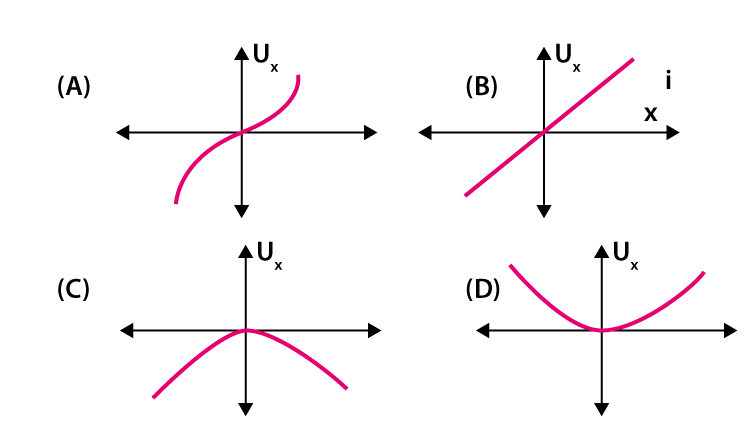## Physics MCQ on Oscillations and Waves for NEET, JEE, Medical and Engineering Exam 2021

MCQ on Oscillations and Waves: Previous trends reveal that NEET/JEE aspirants find Physics as the most challenging section amongst the other sections. But most of them fail to decipher the reason behind this. This particularly can be as the candidates taking up this examination are predominantly medical aspirants with their subject of interest being Biology. Also, sections like Physics are more on theories, laws, numerical as opposed to Biology which is more of fact-based, life sciences and comprising of substantial explanations.In this post we are providing you MCQ on Oscillations and Waves, which will be beneficial for you in upcoming NEET, AIIMS, JEE, WBJEE, Medical and Engineering Exams.

## MCQ on Oscillations and Waves

Q1. On which of the following does the energy of a simple harmonic motion depend ?
a) ⍵
b) 1/ω^2
c) 1/a^2
d) a^2

(d) a^2

Q2. What is the phase difference between the prongs of the tuning fork?
a) 5π
b) π
c) 2π
d) 3π

(b) π

Q3. Calculate the amplitude for a SHM using the equation x = 3sin2pt + 4cos3pt.
a) 3
b) 5
c) 4
d) 7

(b) 5

Q4. The observer hears a sound from the source which is moving away from the observer who is stationary. Determine the frequency of the sound.
a) It is halved
b) It remains the same
c) It reaches infinity
d) It gets doubled

(a) It is halved

Q5. What is the time taken by a particle executing SHM with a time period T sec from a positive extreme position to half of the amplitude?
a) 2T/12 sec
b) T/12 sec
c) 6T/12 sec
d) 3T/12 sec

(a) 2T/12 sec

Q6. What is the change in the phase if a wave is reflected from a denser medium ?
a) 3π
b) 0
c) π
d) 2π

(c) π

Q7. Which of the following graph depicts the variation in the potential energy for a particle executing SHM at the origin x = 0?(d)

Q8. What is the constant of propotionality of an oscillator if the damping force is directly propotional to the velocity ?
a) kg.s^-1
b) kg.m.s^-1
c) kg.s
d) kg.m.s^-2

(a) kg.s^-1

Q9. Which of the following does not exhibit polarization ?
a) Longitudinal wave in a gas
b) Transverse wave in a gas
c) Neither (a) nor (b)
d) Both (a) and (b)

(a) Longitudinal wave in a gas

Q10. Which of the following graph represents the variation in the potential energy as a function of time t and displacement x if the displacement of the particle is x = A cos⍵ta) I,IV
b) II, III
c) I,III
d) II,IV

(c) I,III